• 利用Qt实现N皇后算法，可以单步显示每次的结果。
• ## N皇后算法

千次阅读 2009-11-12 14:07:00
const int N=16;// 在这里设置N值 int Validate(int row,int col,int ExistsTbl[]) { int i; for(i=0;i;i++) { if(row==ExistsTbl[i])//是否同行 { return 0; } else if(r
#include "stdio.h"

const int N=16;// 在这里设置N值
int Validate(int row,int col,int ExistsTbl[])
{
int i;
for(i=0;i<col;i++)
{
if(row==ExistsTbl[i])//是否同行
{
return 0;
}
else if(row-col==ExistsTbl[i]-i||row+col==ExistsTbl[i]+i)//对角线
return 0;
}
return 1;

}
int place_queue(int col,int ExistsTbl[])
{
int row=0,flag=0;
if(col==N)
{
flag=1;
}
else
{
while(row<N&&flag==0)
{
if(Validate(row,col,ExistsTbl))
{
ExistsTbl[col]=row;
flag=place_queue(col+1,ExistsTbl);
}
else
row++;
if(flag==0)//回朔
row++;

}
}
return flag;
}
void main()
{
int ExistsTbl,i,j;

if(place_queue(0,ExistsTbl)==1)
{
printf(" ");
for(i=1;i<=N;i++)
{
printf("%3d",i);
}
printf("/n");
for(i=1;i<=N;i++)
{
printf("%d",i);
for(j=0;j<N;j++)
if(ExistsTbl[j]==i-1)
printf("%3c",'Q');
else
printf("%3c",'*');
printf("/n");
}

}
}  参考文献http://www.cnblogs.com/jillzhang/archive/2007/10/21/922830.html
展开全文include c
• 利用Qt实现的N皇后算法，理论可以实现任意皇后的解法，可以单步演示每种解法。
• N queensN皇后算法N queensN皇后算法的完整源码（定义，实现，main函数测试） N queensN皇后算法的完整源码（定义，实现，main函数测试） #include <iostream> #include <array> /** * @namespace ...
N queensN皇后算法N queensN皇后算法的完整源码（定义，实现，main函数测试）
N queensN皇后算法的完整源码（定义，实现，main函数测试）
#include <iostream>
#include <array>

/**
* @namespace backtracking
* @brief Backtracking algorithms
*/
namespace backtracking {
/**
* @namespace n_queens
* @brief Functions for [Eight Queens](https://en.wikipedia.org/wiki/Eight_queens_puzzle) puzzle.
*/
namespace n_queens {
/**
* Utility function to print matrix
* @tparam n number of matrix size
* @param board matrix where numbers are saved
*/
template <size_t n>
void printSolution(const std::array<std::array<int, n>, n> &board) {
std::cout << "\n";
for (int i = 0; i < n; i++) {
for (int j = 0; j < n; j++) {
std::cout << "" << board[i][j] << " ";
}
std::cout << "\n";
}
}

/**
* Check if a queen can be placed on matrix
* @tparam n number of matrix size
* @param board matrix where numbers are saved
* @param row current index in rows
* @param col current index in columns
* @returns true if queen can be placed on matrix
* @returns false if queen can't be placed on matrix
*/
template <size_t n>
bool isSafe(const std::array<std::array<int, n>, n> &board, const int &row,
const int &col) {
int i = 0, j = 0;

// Check this row on left side
for (i = 0; i < col; i++) {
if (board[row][i]) {
return false;
}
}

// Check upper diagonal on left side
for (i = row, j = col; i >= 0 && j >= 0; i--, j--) {
if (board[i][j]) {
return false;
}
}
// Check lower diagonal on left side
for (i = row, j = col; j >= 0 && i < n; i++, j--) {
if (board[i][j]) {
return false;
}
}
return true;
}

/**
* Solve n queens problem
* @tparam n number of matrix size
* @param board matrix where numbers are saved
* @param col current index in columns
*/
template <size_t n>
void solveNQ(std::array<std::array<int, n>, n> board, const int &col) {
if (col >= n) {
printSolution<n>(board);
return;
}

// Consider this column and try placing
// this queen in all rows one by one
for (int i = 0; i < n; i++) {
// Check if queen can be placed
// on board[i][col]
if (isSafe<n>(board, i, col)) {
// Place this queen in matrix
board[i][col] = 1;

// Recursive to place rest of the queens
solveNQ<n>(board, col + 1);

board[i][col] = 0; // backtrack
}
}
}
} // namespace n_queens
} // namespace backtracking

/**
* Main function
*/
int main() {
const int n = 4;
std::array<std::array<int, n>, n> board = {
std::array<int, n>({0, 0, 0, 0}),
std::array<int, n>({0, 0, 0, 0}),
std::array<int, n>({0, 0, 0, 0}),
std::array<int, n>({0, 0, 0, 0})
};

backtracking::n_queens::solveNQ<n>(board, 0);
return 0;
}



展开全文• 算法设计的N皇后算法，用C++语言编写，控制台程序。
• 实现n皇后算法，解决这个问题，采用的是c++编程语言
• 基于python用递归算法输出N皇后所有可能的情况并记录情况个数
• N 皇后算法 十二个不同的代码 我和同学解决N皇后问题的算法， 语言为C++ 包括有栈实现，和一维数组实现 和一维动态数组实现 和二维数组实现。 还有收录了两个来自于网上的算法也就是我们所参考的算法。 一个为剪枝...
• n皇后算法，，回溯，for循环嵌套递归，矩阵来存储数据
• 本书利用c语言的知识，对N皇后问题的算法进行分析，并在程序设计的过程中，通过对算法的改进，提高程序的运行效率。
• n 皇后问题研究的是如何将 n皇后放置在 n×n 的棋盘上，并且使皇后彼此之间不能相互攻击。 上图为 8 皇后问题的一种解法。 给定一个整数 n，返回所有不同的 n 皇后问题的解决方案。 每一种解法包含一个明确的 n ...
问题描述
n 皇后问题研究的是如何将 n 个皇后放置在 n×n 的棋盘上，并且使皇后彼此之间不能相互攻击。上图为 8 皇后问题的一种解法。
给定一个整数 n，返回所有不同的 n 皇后问题的解决方案。
每一种解法包含一个明确的 n 皇后问题的棋子放置方案，该方案中 ‘Q’ 和 ‘.’ 分别代表了皇后和空位。
示例:
输入: 4
输出: [
[".Q…",  // 解法 1
“…Q”,
“Q…”,
“…Q.”],
["…Q.",  // 解法 2
“Q…”,
“…Q”,
“.Q…”]
]
解释: 4 皇后问题存在两个不同的解法。
解题思路代码
代码思路
一行一行地摆放，在确定一行中的那个皇后应该摆在哪一列时，需要当前列是否合法，如果合法，则将皇后放置在当前位置，并进行递归，回溯。每行都摆满皇后时，则产生了一种解法，将所有解法收集并返回。
判断合法：当前将要摆放’Q’的位置和其他已摆放‘Q’的位置不能在同一列，且不能在同一条45度斜线或135度斜线上。这里判断是否在同一条斜线上可通过当前将要摆放’Q’的位置和其他已摆放‘Q’的位置横坐标之差和纵坐标之差的绝对值是否相等来判断
class Solution {
public List<List<String>> solveNQueens(int n) {
char[][] chs=new char[n][n];
for(int i=0;i<n;i++){
for(int j=0;j<n;j++){
chs[i][j]='.';
}
}
List<List<String>> res=new ArrayList<>();
backTracing(chs,0,n,res);
return res;
}
public void backTracing(char[][] chs,int row,int n,List<List<String>> res){
//每行都摆满皇后时，则产生了一种解法
if(row==n){
return;
}
//一行一行地摆放，在确定一行中的那个皇后应该摆在哪一列时，需要当前列是否合法。
//如果合法，则将皇后放置在当前位置，并进行递归，回溯。
for(int col=0;col<chs.length;col++){
if(isValid(chs,row,col)){
chs[row][col]='Q';
//递归
backTracing(chs,row+1,n,res);
//回溯
chs[row][col]='.';
}
}
}
public List<String> chsToList(char[][] chs){
List<String> list=new ArrayList<>();
for(int i=0;i<chs.length;i++){
}
return list;
}
//判断合法：当前将要摆放'Q'的位置和其他已摆放‘Q’的位置不能在同一列，且不能在同一条45度斜线或135度斜线上。
//这里判断是否在同一条斜线上可通过当前将要摆放'Q'的位置和其他已摆放‘Q’的位置横坐标之差和纵坐标之差的绝对值是否相等来判断。
public boolean isValid(char[][] chs,int x,int y){
for(int i=0;i<=x;i++){
for(int j=0;j<chs.length;j++){
if(chs[i][j]=='Q'&&(j==y||Math.abs(x-i)==Math.abs(y-j))){
return false;
}
}
}
return true;
}
}




展开全文• 基于N皇后算法的CPU速度测试程序，只写了两个线程，更多线程原理一样
• ## N皇后算法—优化版

万次阅读 2017-11-01 21:42:34
N皇后问题 【题目描述】 N皇后问题 即在NXN格的国际象棋上摆放N个皇后，使其不能互相攻击，即任意两个皇后都不能处于同一行、同一列或同一斜线上，请问有多少中摆法，并将每种摆法打印出来。图1所示即是摆法的一...
N皇后问题

【题目描述】 N皇后问题

即在NXN格的国际象棋上摆放N个皇后，使其不能互相攻击，即任意两个皇后都不能处于同一行、同一列或同一斜线上，请问有多少中摆法，并将每种摆法打印出来。图1所示即是摆法的一种。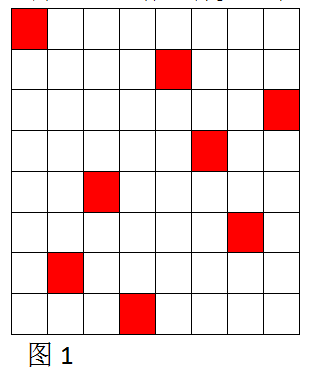【输入格式】

输入一个整数，即N(14>N>3)。

【输出格式】

输出所有摆法，每个摆法占一行

【输入样例】

4

【输出格式】

2413

3142

【输出说明】

N=4的棋盘输出的两种方案即图2所示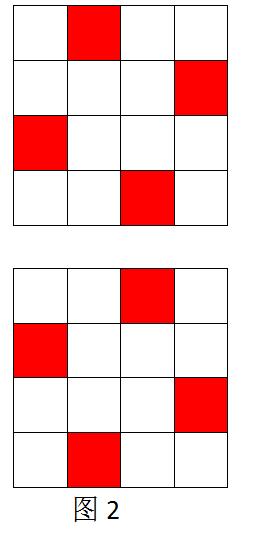递归算法1★

若想遍历所有摆法而无一遗漏，可以逐行从上至下、从左至右尝试棋子的摆放。以N=4的棋盘为例，其遍历过程如图3所示。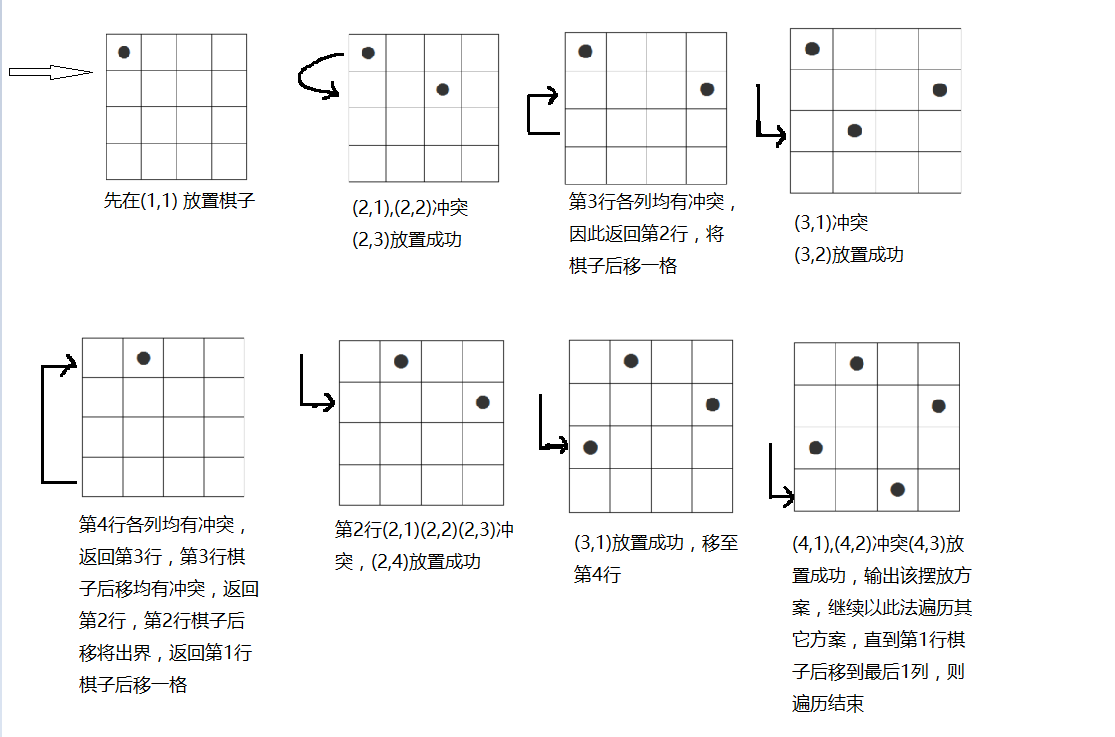Tips:棋盘坐标上一般会想到用二维数组来表示，如定义二维数组a,则a=1代表棋盘第一行第一列有棋子，a=0代表棋盘第4行第5列无棋子……但实际上只用一个一维数组可以解决该问题。

例如，使用a来表示棋盘坐标时，假设a=7，即表示第1行第7列有棋子，a=2即表示第2行第2列有棋子，而且这种方法无须再判断两皇后是否在同一行。

可以定义一个Try(x,y)函数判断棋盘(x,y)处是否可以放皇后：

(1)不在同一列

(2)不在对角线上，即有两棋子坐标分别为(X1,Y1),(X2,Y2)，则|X1-X2|!=|Y1-Y2|

源码：

//N皇后问题——递归算法
#include<iostream>
#include<stdio.h>
#include<stdlib.h>
using namespace std;
int n,a;

void print()				//打印棋子
{int i=1;
for(;i<=n;i++)
cout<<a[i];
cout<<" ";

if(i%7==0)
cout<<endl;
}

void printGraph()			//图形化打印布局
{
system("color F0");  	//DOs命令改变背景色和前景色，颜色为16进制数0~F
for(int i=1;i<=n;++i)
{
for(int j=1;j<=n;++j)
if(a[i] == j)
cout<<"O";
else
cout<<"▅";
cout<<endl;
}
cout<<endl;
}

int Try(int x,int y)			//测试x行y列可否摆放棋子，成功返回1，否则返回0
{
int j=1;
while(j<x)					//与数组中已放好的数比较
{
if((a[j] == y) || (abs(x-j) == abs(a[j]-y)))
return 0;
++j;					//右移一格继续尝试
}
return 1;
}

void place(int x)				//递归函数
{
int y;
if(x>n)						//棋子第n行已摆好，则打印成功方案
{
print();
//printGraph();			//打印图形化布局
}
else
{
for(y=1;y<=n;++y)		//该行棋子依次从左向右移
if(Try(x,y))		//如果可以摆放
{
a[x]=y;			//给a[x]赋值
place(x+1);		//继续下一行的递归
}
}
}

int main()
{
cin>>n;
place(1);			//从第1行开始递归
return 0;
}

该程序中有个用于调试的printGraph()函数，选用它可图形化显示布局方案，如图所示：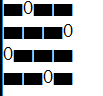为了深入理解递归调用的过程，设N=4，则可将place(4)到place(4)的过程展开，如图所示：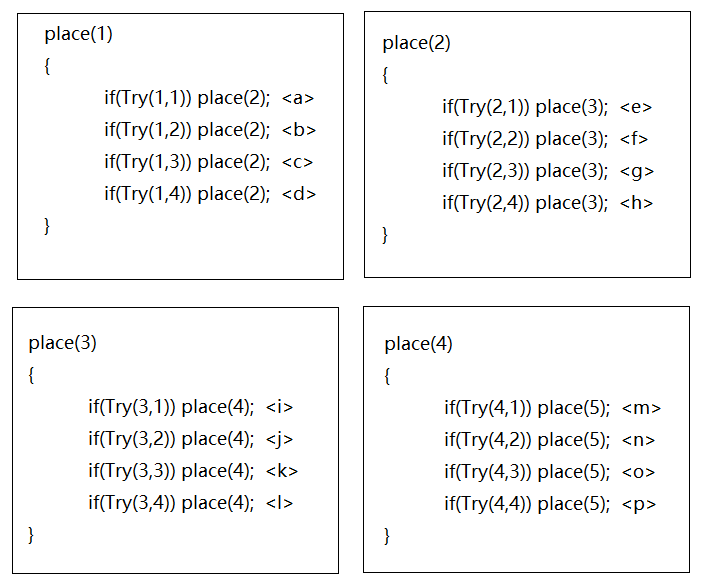运行过程如图下图所示。

模拟该程序运行过程时会发现，通过程序不断的进进退退，最后就打印除了全部的布局。

由此可知，递归中常常隐含回溯，回溯即选择一条路走下去，发现走不通，就返回去再选一条路走。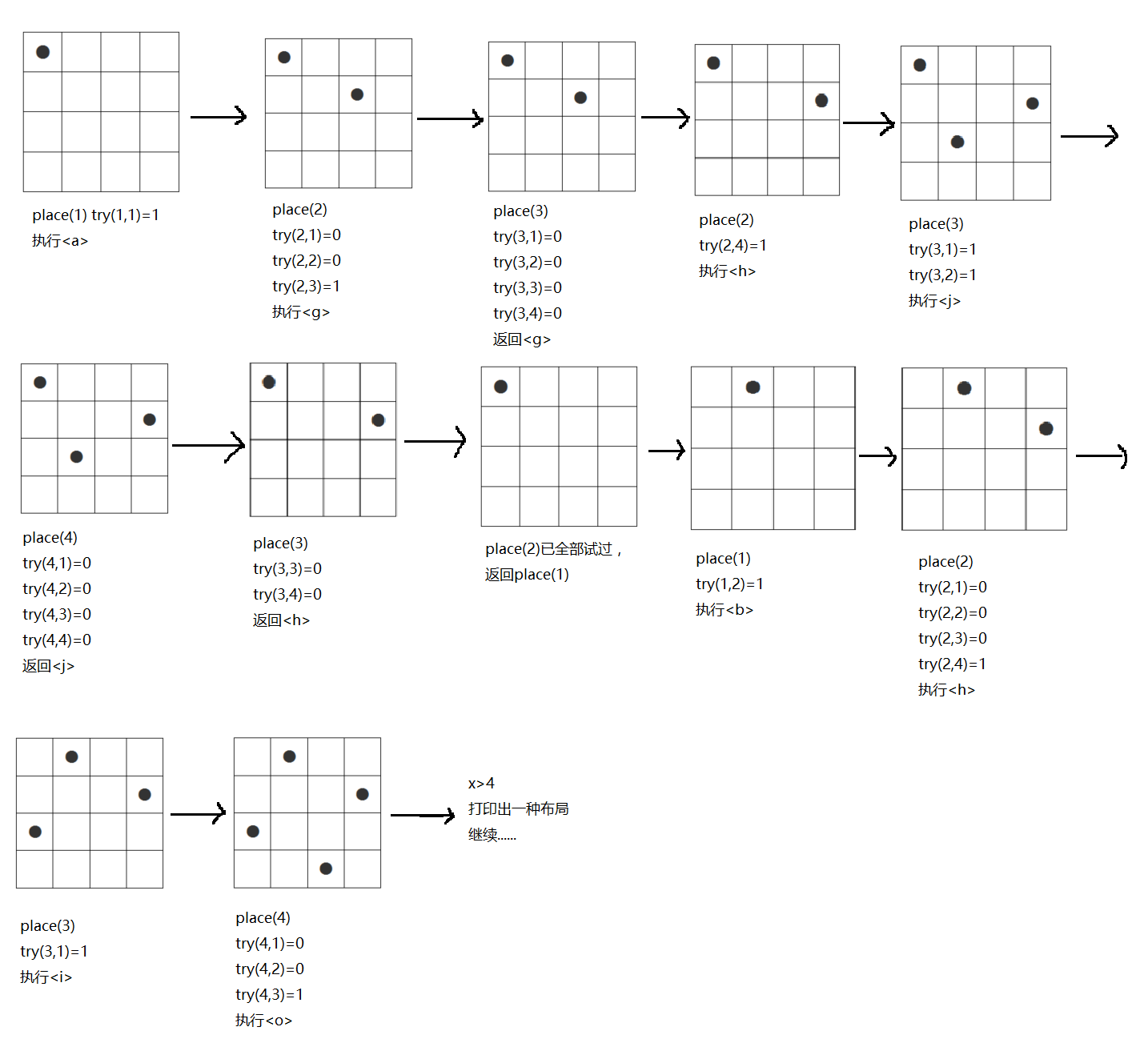以下为八皇后问题的解决，共92种摆法：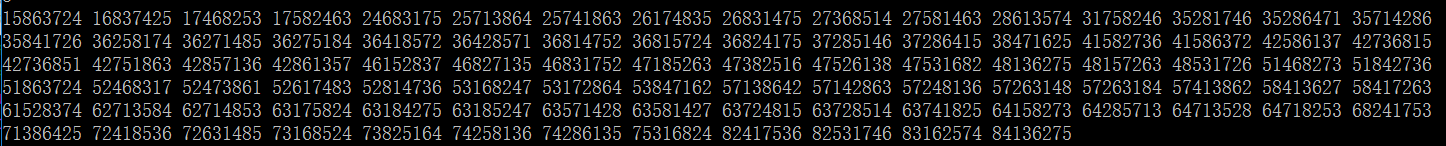改程序当N>13时，速度将慢的难以忍受，并且考虑到输出方案浪费了大量的时间，此后的优化算法只需要输出方案数即可。

递归算法2★

以N=4为例，下图左是正斜线，右是反斜线，即有2xN-1条、反斜线。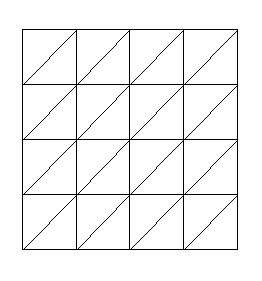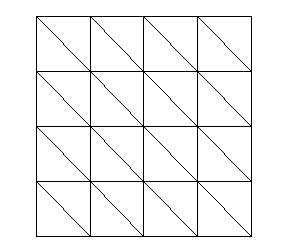1号正斜线所占据的棋盘单元为(1,1)

2号正斜线所占据的棋盘单元为(1,2)(2,1)

3号正斜线所占据的棋盘单元为(1,3)(2,2)(3,1)

4号正斜线所占据的棋盘单元为(1,4)(2,3)(3,2)(4,1)

5号正斜线所占据的棋盘单元为(2,4)(3,3)(4,2)

6号正斜线所占据的棋盘单元为(3,4)(4,2)

7号正斜线所占据的棋盘单元为(4,4)

可以发现规律如下：

(1)   同一正斜线所占据的棋盘单元行列之和相等，如上面7条正斜线分别为2、3、4、5、6、7、8.

(2)   同一反斜线所占据的棋盘单元行列之差相等。

故可以定义bool数组x1[ ]用来记录行列之和为i的正斜线是否已经被占据，bool数组x2[ ]用来记录行列之差为i的反斜线是否已经被占据。考虑到行列之差可能为负数，棋盘坐标(x,y)对应x2[ x-y+n]。

再定义一个bool数组y[ ]判断列冲突，如果y[i]=1，说明前面已有皇后放到在这一列，再放会发生列冲突，y[i]=0，说明当前第i列还没有放置皇后。

参考代码：

//N皇后——递归算法2
#include<iostream>
#include<cstdio>
#include<cstdlib>

using namespace std;

int n,num;

int a={0};
bool y,x1,x2;

void place(int x)   					//递归函数
{
if(x>n)
++num;							//找到一个摆法，计数器加1
else
{
for(int i=1;i<=n;++i)
{
if(y[i] == 0 && x1[x+i] ==0 && x2[x-i+n] == 0)		//如果列，正斜线，反斜线无冲突
{
a[x] = i;						//给a[x]赋值
y[i] = 1;						//列坐标做标记
x1[x+i] = 1;					//正斜线做标记
x2[x-i+n] = 1;					//反斜线做标记
place(x+1);						//继续下一行的递归
y[i] = 0;						//恢复
x1[x+i] = 0;					//恢复正斜线标记为0
x2[x-i+n] = 0;					//恢复反斜线标记为0
}
}
}
}

int main()
{
cin>>n;
place(1);
cout<<num<<endl;
}

优化后的程序提高了很多，但是当N>=14时候，速度还是较慢，我们还可以再次基础上继续优化，这里我就不继续写了，希望大家能够自己优化。


展开全文c语言 优化
• N皇后算法的运算过程用图形的方式显示。 本压缩包包含编译好的exe可运行文件和源代码。可以重新编译和修改。 本程序在Microsoft Visual C++ 2008 Express Edition编译并调试通过。 想运行本程序需要您的机器上装有...
• 8皇后以及N皇后算法探究，回溯算法的JAVA实现，递归方案 8皇后以及N皇后算法探究，回溯算法的JAVA实现，非递归，数据结构“栈”实现 研究了递归方法实现回溯，解决N皇后问题，下面我们来探讨一下非递归方案 实验...
• 优化后的 8皇后算法 很快 绝对超值，不下后悔，
• # /uer/bin/env python# *_*coding=utf-8 *_*x=[0 for i in range(1000)] #存放结果的列表sum1=0def Place(t): global x global n for i in range(1,t): if (abs(x[i]-x[t])==abs(i-t)) |(x[i]==x[t]): return ...python
• 皇后问题，是一个古老而著名的问题，是回溯算法的典型案例。该问题是国际西洋棋棋手马克斯·贝瑟尔于1848年提出：在8×8格的国际象棋上摆放八个皇后，使其不能互相攻击，即任意两个皇后都不能处于同一行、同一列或...
• 皇后问题，是一个古老而著名的问题，是回溯算法的典型案例。该问题是国际西洋棋棋手马克斯·贝瑟尔于1848年提出：在8×8格的国际象棋上摆放八个皇后，使其不能互相攻击，即任意两个皇后都不能处于同一行、同一列或...
• 用C#做的N皇后算法简单，默认是八皇后，能够查看每一种解法的情况，用画图的形式表现，界面上用了皮肤控件，感觉还算不错。
• 皇后问题，是一个古老而著名的问题，是回溯算法的典型案例。该问题是国际西洋棋棋手马克斯·贝瑟尔于1848年提出：在8×8格的国际象棋上摆放八个皇后，使其不能互相攻击，即任意两个皇后都不能处于同一行、同一列或...
• 求最大公约数（欧几里得算法尾递归版）+N皇后算法（位运算版） 求最大公约数思路：沿用欧几里得算法直接出最简洁代码，数学证明重点在于gcd（a, b) = gcd（b, amodb)上。 /* 欧几里得算法求最大公约数*/ int ...笔试 gcd
• java原文参考这位大神，他的博客中有关于N皇后问题的极限算法 https://www.cnblogs.com/newflydd/p/5091646.html 我只是搬运翻译的js实现 console.log使用node.js到时候替换即可。 //定义数组克隆方法 Array....js javascript
• 算法实验、利用MFC模拟算法中的N皇后，其中使用STL中的map、vector实现存储所有可行性。
• 《8皇后以及N皇后算法探究，回溯算法的JAVA实现，递归方案》 是使用递归方法实现回溯算法的，在第一次使用二维矩阵的情况下，又做了一次改一维的优化 但是算法效率仍然差强人意，因为使用递归函数的缘故 下面提供...
• N皇后描述： 任何一行一列 45度斜线上都只能有一个皇后 要求： 以四皇后为例打印出符合条件的所有结果 #include #include using namespace std; const int N = 4; //number of the box int row[N+1] = {0}; ......